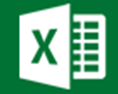AJP Excel Information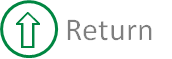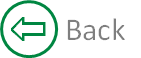##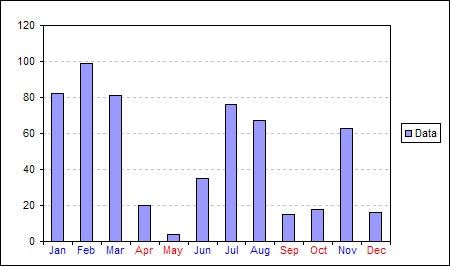##### Here is the data and formula used to build the chart. The actual data for the column chart is in the range C3:C14. The formula in columns D and E test the Data value and either output a zero or #N/A depending on whether a red or blue label should be displayed.   B C D E 2 Data Red Labels Blue Labels 3 Jan 82 =IF(C3<25,0,NA()) =IF(C3>=25,0,NA()) 4 Feb 99 =IF(C4<25,0,NA()) =IF(C4>=25,0,NA()) 5 Mar 81 =IF(C5<25,0,NA()) =IF(C5>=25,0,NA()) 6 Apr 20 =IF(C6<25,0,NA()) =IF(C6>=25,0,NA()) 7 May 4 =IF(C7<25,0,NA()) =IF(C7>=25,0,NA()) 8 Jun 35 =IF(C8<25,0,NA()) =IF(C8>=25,0,NA()) 9 Jul 76 =IF(C9<25,0,NA()) =IF(C9>=25,0,NA()) 10 Aug 67 =IF(C10<25,0,NA()) =IF(C10>=25,0,NA()) 11 Sep 15 =IF(C11<25,0,NA()) =IF(C11>=25,0,NA()) 12 Oct 18 =IF(C12<25,0,NA()) =IF(C12>=25,0,NA()) 13 Nov 63 =IF(C13<25,0,NA()) =IF(C13>=25,0,NA()) 14 Dec 16 =IF(C14<25,0,NA()) =IF(C14>=25,0,NA())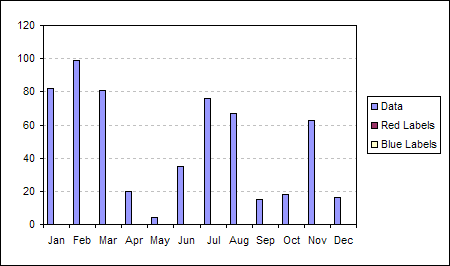##### Select the range B2:E14 and use the chart wizard to build a standard Clustered Column chart.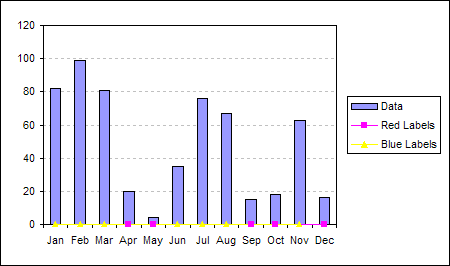##### Repeat for the 'Blue Label' series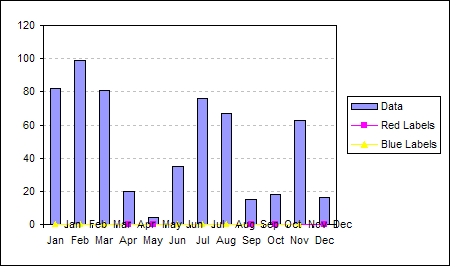##### Double click the 'Red Labels' series and on the Data Labels tab of the Format Data Series dialog check the Category name option.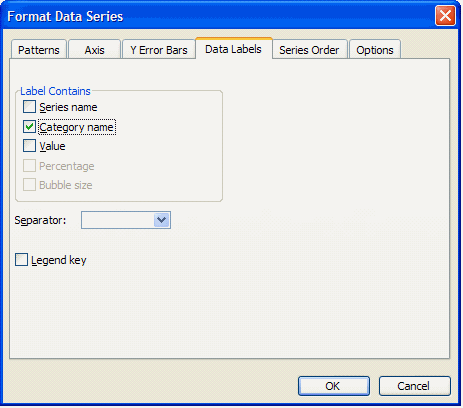##### Repeat the application of data labels for the 'Blue Labels' series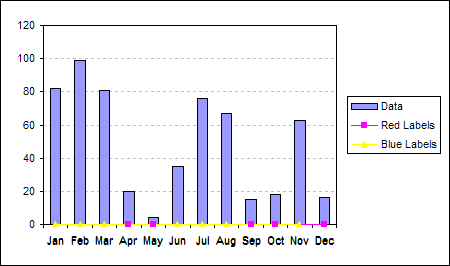##### Double click the 'Red Labels' data labels and on the Alignment tab of the Format Data Labels dialog set the Label Position to Below.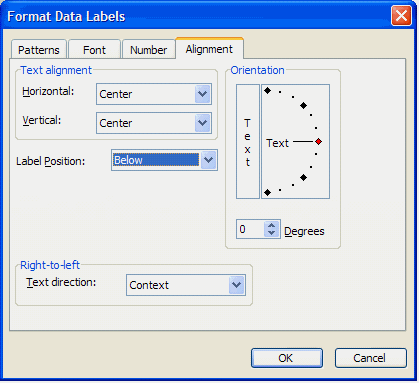##### Repeat data label label position for the 'Blue Labels' series.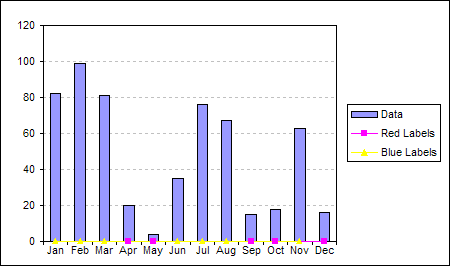##### This will clear the built-in axis labels.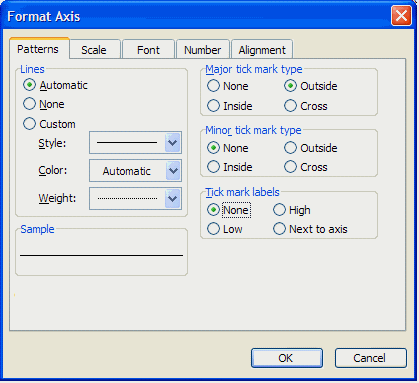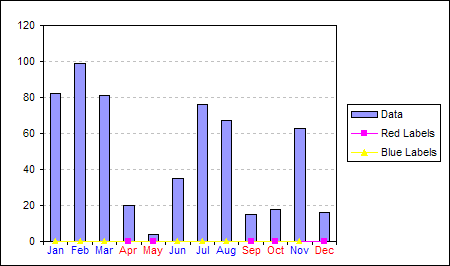##### Double click the 'Red Labels' data labels and on the Font tab of the Format Data Labels dialog set the Font Colour to Red.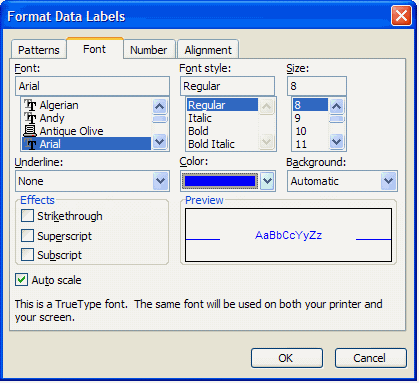##### Repeat for the 'Blue Labels' data labels. Setting the Font Colour to Blue.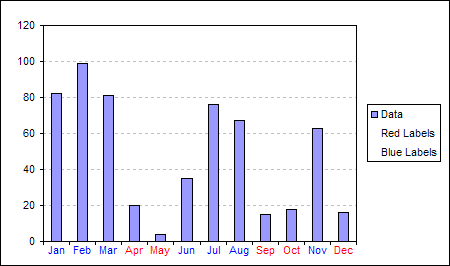##### Double click the 'Red Labels' data series and on the Patterns tab of the Format Data Series set the Line and Marker to None.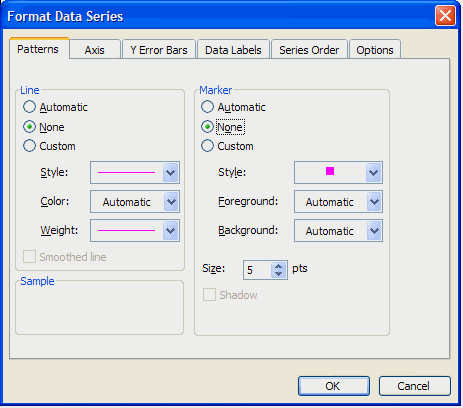##### For a explanation of how to remove the extra series from the legend see, Delete a single entry from the legend.

Created August 2004
Last updated 5th August 2014

Microsoft® and Microsoft® Excel are registered trademarks of the Microsoft Corporation.
andypope.info is not associated with Microsoft. Copyright ©2007-2016 Andy Pope